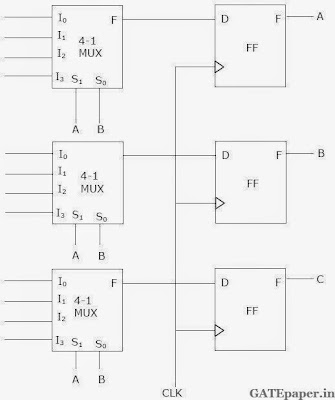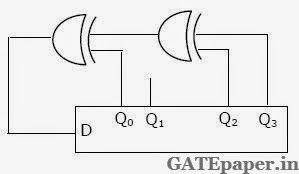### GATE 1996 ECE Video Solutions on Digital Circuits (Digital Electronics)

1. Schottky clamping is resorted in TTL gates
a. To reduce propagation delay
b. To increase noise margins
c. To increase packing density
d. To increase fan-out

2. A pulse train can be delayed by a finite number of clock periods using a
a. Serial In Serial Out shift register
b. Serial In Parallel Out shift register
c. Parallel In serial Out shift register
d. Parallel In parallel Out shift register
Solution :

3. A 12 bit ADC is operating with a 1 µsec clock period and the total conversion time is seen to be 14 µsec. the ADC must be of the
a. Flash type
b. Counting type
c. Integrating type
d. Successive Approximation type

4. The total number of memory accesses involved (inclusive of the op-code fetch), when an 8085 processor executes the instruction LDA 2003 is
a. 1
b. 2
c. 3
d. 4
Solution :

5. A Dynamic RAM cell which holds 5 volts has to be refreshed every 20 ms, so that the stored voltage does not fall by more than 0.5 volts. If the cell has a constant discharge current of 0.1 pA, the storage capacitance of the cell is

6. A 10 bit ADC with full scale output voltage of 10.24 volts is designed to have a ±LSB/2 accuracy. If the ADC is calibrated at 25oC and the operating temperature ranges from 0oC to 50oC, then the maximum net temperature coefficient of the ADC should not exceed
a. ± 200 µV/oC
b. ± 400 µV/oC
c. ± 600 µV/oC
d. ± 800 µV/oC
Solution :

7. A memory system of size 26K bytes is required to be designed using memory chips which have 12 address lines and 4 data lines each. The number of such chips required to design the memory system is
a. 2
b. 4
c. 8
d. 13

8. The following sequence of instructions are executed by an 8085 microprocessor:
1000 LXI SP, 27FFH
1003 CALL 1006
1006 POP H
The contents of the stack pointer (SP) and the HL register pair on completion of execution of these instructions are
a. SP = 27FF, HL = 1003
b. SP = 27FD, HL = 1003
c. SP = 27FF, HL = 1006
d. SP = 27FD, HL = 1006
Solution :

9. Given the Boolean function F in three variables R, S and T as
F = R’ST’ + RS’T + RST
a. Express F in the minimum sum of products form
b. Express F in the minimum product of sums form
c. Assuming that both true and complement forms of the input variables are available, draw a circuit to implement F using the minimum number of 2 input NAND gates only.

10. A state machine is required to cycle through the following sequence of states:
ABC : 000 -> 010 -> 111 -> 100 -> 011 -> 101.One possible implementation of the state machine is shown in figure. Specify what signals should be applied to each of the multiplexer inputs.

11. A 4 bit shift register, which shifts 1 bit to the right at every clock pulse, is initialized to values 1000 for (Q0Q1Q2Q3). The D input is derived from Q0, Q2 and Q3 through two XOR gates as shown in figure.a. Write the 4 bit values (Q0Q1Q2Q3) after each clock pulse till the pattern (1000) reappears on (Q0Q1Q2Q3).
b. To what values should the shift register be initialized so that the pattern (1001) occurs after the first clock pulse?

12. It is desired to generate the following three Boolean functions using an OR array as shown in figure, where P1 to P5 are the product terms in one or more of the variables a, a’, b, b’, c and c’.Write down the terms P1, P2, P3, P4 and P5.